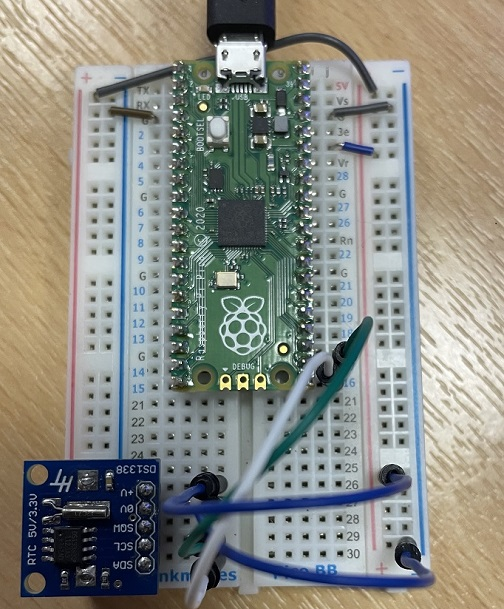# Raspberry Pi Pico RTC DS1388

An RTC is a real time clock. These are integrated circuits which, when combined with a few other parts can be used to keep time. You tend to get them mounted on PCBs ready to go as they are popular components for makers. Many of the RTCs that are easiest to use need a 5V supply. The DS1388 is a board made by the UK company Hobbytronics. The board no longer appears to be on sale. I have included this here because I got it to work first try and as the code may illustrate some concepts that could be reused with other components.

There are 4 connections to make. 0V goes to GND, and +V goes to 3V. SDA connects to GP16 and SCL connects to GP17.I have written the code for this as a separate class. In Thonny, save this file to the Pico with the name ds1338.py.

```from machine import Pin, I2C

def bcd2bin(value):
return (value or 0) - 6 * ((value or 0) >> 4)

def bin2bcd(value):
return (value or 0) + 6 * ((value or 0) // 10)

class rtc:
def __init__(self, d, c):
self.i2c=I2C(0,sda=Pin(d), scl=Pin(c))

def get_time(self):
s = bcd2bin(buf & 0x7F)
m = bcd2bin(buf)
h = bcd2bin(buf)
w = bcd2bin(buf)
dd = bcd2bin(buf)
mm = bcd2bin(buf)
yy = bcd2bin(buf) + 2000
return [s,m,h,w,dd,mm,yy]

def set_time(self,s,m,h,w,dd,mm,yy):
tm = bytes([bin2bcd(i) for i in [s,m,h,w,dd,mm,yy-2000]])
self.i2c.writeto_mem(int(0x68), int(0),tm)
```

In your main.py file, you need something like this, depending on what you want to do with the time readings. The first time you run the code, you need to make sure to set the time on the RTC. I have commented out that line in the following code that goes in the main.py file.

```from ds1338 import rtc
from time import sleep

myclock = rtc(16, 17)

# s,m,h,w,dd,mm,yy
#myclock.set_time(0,19,10,4,3,2,2022)

while True:
t = myclock.get_time()
hr = str(t)
mins = str(t)
secs = str(t)
if len(hr)<2: hr = "0" + hr
if len(mins)<2: mins = "0" + mins
if len(secs)<2: secs = "0" + secs
mytime = str(hr) + ":" + str(mins) + ":" + str(secs)
print(mytime)
sleep(1)
```